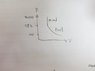# Polytropic process question

• Joon
In summary, in this problem, we are given initial conditions of an ideal gas in a cylinder and asked to calculate the pressure and temperature after undergoing a two-stage process of compression and constant volume heating. Using the polytropic equation and the ideal gas law, we can solve for the pressure and temperature at each stage, as well as calculate the work done in the first process. Drawing a P-V diagram, we can see that the gas follows a polytropic curve for the first stage and then remains at constant volume for the second stage, resulting in a steep rise in pressure.f

## Homework Statement

At the start of a compression, a cylinder contains 0.04 m^2 of some ideal gas at a pressure of 100kPa and a temperature of 110 degrees. Compression takes place according to the law:

P(V)^1.27 = constant

The gas undergoes a two stage process: compression from 0.04 m^3 to 0.01 m^3, followed by constant volume heating to raise the pressure to 4000 kPa.

a. Calculate the pressure and temperature of the gas after the first process.
b. Calculate the temperature of the gas after the second process.
c. Calculate the work done.
d. Draw the P-V diagram, clearly indicating the two stages.

## Homework Equations

P(V)^1.27 = constant

## The Attempt at a Solution

I solved question (a) by using the equation P1(V1)^1.27 = P2(V2)^1.27 and found that after the first process the pressure is 582 kPa, V= 0.01 m^3 and T= 556 K.
For question (b), do I only need to use the equation T2/T1 = (P2/P1)^(0.27/1) to get the temperature after the second process? It says constant volume heating then does it mean volume is unchanging?

For question (c), is it work done in the first process or the second or both combined?
W= (P2V2-P1V1) / 1-1.27 is the equation I am going to use.

Thank you.

does it mean volume is unchanging?
yes.
For question (c), is it work done in the first process or the second or both combined?
Hint: what is the work done when volume is constant?
Draw the P-V diagram, clearly indicating the two stages.
try to think what the slope of a polytropic process is and what its graph looks like for the first process. For the second process, volume is constant and temperature rises, what does that imply?
(b), do I only need to use the equation T2/T1 = (P2/P1)^(0.27/1)
from ##PV=nRT## substitute T into the equation already given to get it in terms of T and P; you can then use it.

P.S, this is the first time I have answered on this forum, so pardon if I've made any mistakes

For question (b) then, I don't need to use the polytropic equation P(V)^n = constant, right?
Using PV=nRT, should I say (P1) / T1 = (P2) / T2 ?

So for question (c), it means the work done in the second process, right? As dW = PdV, I guess when volume is unchanging the work done is 0.
For question (d), I think volume being constant as temperature rises implies that pressure also rises. But I'm not sure how to draw the diagram, it would be nice if you could help.

I don't need to use the polytropic equation P(V)^n = constant, right?
Using PV=nRT, should I say (P1) / T1 = (P2) / T2 ?
Your expression is wrong, I am writing P(v)^n as P(v)^x to avoid confusion between moles and variable. you need to use PV=nRT to write (v)^x as (nRT/P)^x, now substitute V^n in the original equation to get the correct expression (note that (nR)^x is constant)
So for question (c), it means the work done in the second process, right? As dW = PdV, I guess when volume is unchanging the work done is 0.
till otherwise stated, its always the work combined of both processes, and you're right constant volume implies 0 work.
For question (d), I think volume being constant as temperature rises implies that pressure also rises. But I'm not sure how to draw the diagram, it would be nice if you could help.
x axis represents volume, volume is constant so x is constant while P is rising therefore y is rising. This implies which line?

I calculated as follows after changing V into nRT/P . Please check if the steps are correct.

P2 x (nRT2 / P2)^1.27 = P1 x (nRT1 / P1)^1.27
4000 x (T2)^1.27 / 4000^1.27 = 582 X 556^1.27 / 582^1.27
T2 = 83.76 K

For question (c), as the work done in the second process is 0, I just need to consider the work done in the first process.
W = (P2V2 -P1V1) / 1-n = (582x10^3 x0.01 - 100 x 10^3 x 0.04) / 1-1.27 = -6740 J

Last edited:
For the diagram, would it be as simple as this?

#### Attachments

•38479603_296383444451740_5111468328753299456_n.jpg
15.6 KB · Views: 423
P2 x (nRT2 / P2)^1.27 = P1 x (nRT1 / P1)^1.27
correct.
For question (c), as the work done in the second process is 0, I just need to consider the work done in the first process
also correct.
For the diagram, would it be as simple as this?
yes, well done! :D

•Joon